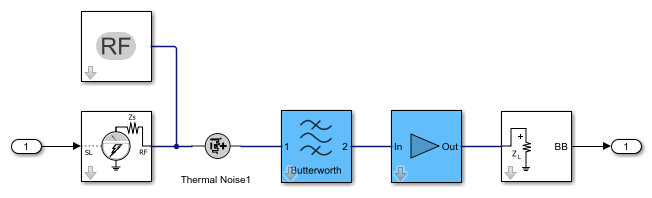# open_system

Open RF Blockset model created using `rfsystem`

Since R2021a

## Syntax

``open_system(rfs)``

## Description

example

````open_system(rfs)` opens the RF Blockset™ model created using the `rfsystem` System object™ `rfs`. ```

## Examples

collapse all

Create a fifth-order bandpass RF filter and an amplifier with the gain of `3` dB.

```f1 = rffilter('ResponseType','Bandpass','FilterOrder',5,'PassbandFrequency',[4.85 5.15]*1e9); a1 = amplifier('Gain',3,'NF',1.53,'OIP3',35); ```

Create an `rfbudget` object using the elements at an input frequency of `5` GHz, an available input power of `-30` dBm, and a bandwidth of `200` MHz.

```rfb = rfbudget([f1 a1],5e9,-30,200e6); ```

Create an RF system using the `rfbudget` object and name the RF Blockset model.

```rfs = rfsystem(rfb,'ModelName','RFSystem'); ```

Open the RF Blockset model.

```open_system(rfs) ```Save and close the RF Blockset model.

```save_system(rfs); close_system(rfs) ```

## Input Arguments

collapse all

RF system, specified as an `rfsystem` object

## Version History

Introduced in R2021a